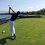# Overview

All power sums have a closed polynomial forms for integral powers. For example,

$1^2+2^2+3^2+\cdots+n^2=\displaystyle \sum_{k=1}^n k^2=\frac{n^3}{3}+\frac{n^2}{2}+\frac{n}{6}$

More generally

$1^m+2^m+3^m+\cdots+n^m=\displaystyle \sum_{k=1}^n k^m=\displaystyle \sum_{i=1}^{m+1} a_i n^i$

In the case of $m=2$, $a_1=\frac{1}{6}$, $a_2=\frac{1}{2}$, and $a_3=\frac{1}{3}$.

# Solving for the General Case

To solve for the closed form solution of every power sum we use the following process.

Take the bottom left half of Pascal's infinite matrix. Remove the top left-bottom right diagonal of 1's. Remove the top row and right most column of 0's. Invert the matrix. Now, the $j$th row from the right yields the coefficients, $a_i$ of the sum of (j-1)th powers. A full proof and explanation is given in this document.

# Table of Coefficients for Closed form Solutions

This table contains the coefficients of the closed form solutions for the first 61 power sums. As an example, the 3rd column from the right reads:

$\begin{array}{c}\\ \frac{1}{3}\\ \frac{1}{2}\\ \frac{1}{6} \end{array}$

This means $a_3=\frac{1}{3}$, $a_2=\frac{1}{2}$, and $a_1=\frac{1}{6}$. Thus the closed form solution is $a_3n^3+a_2n^2+a_1n=\frac{1}{3}n^3+\frac{1}{2}n^2+\frac{1}{6}n$.

# How it was made:

I used the code below for CS50 to generate the bottom 61 rows and columns of Pascal's matrix. After eliminating the unnecessary rows and columns, I then inverted the matrix on this website (huge thanks to whomever made this). I had to fill in 455 0's (probably due to the range of a long double) by hand but don't worry, all numbers in the giant table linked above are accurate.

  1 2 3 4 5 6 7 8 9 10 11 12 13 14 15 16 17 18 19 20 21 22 23 24 25 26 27 28 29 30 31 32 33 34 35 36 37 38 39 40 41 #includeNote by Trevor Arashiro
4 years, 3 months ago

This discussion board is a place to discuss our Daily Challenges and the math and science related to those challenges. Explanations are more than just a solution — they should explain the steps and thinking strategies that you used to obtain the solution. Comments should further the discussion of math and science.

When posting on Brilliant:

• Use the emojis to react to an explanation, whether you're congratulating a job well done , or just really confused .
• Ask specific questions about the challenge or the steps in somebody's explanation. Well-posed questions can add a lot to the discussion, but posting "I don't understand!" doesn't help anyone.
• Try to contribute something new to the discussion, whether it is an extension, generalization or other idea related to the challenge.
• Stay on topic — we're all here to learn more about math and science, not to hear about your favorite get-rich-quick scheme or current world events.

MarkdownAppears as
*italics* or _italics_ italics
**bold** or __bold__ bold
- bulleted- list
• bulleted
• list
1. numbered2. list
1. numbered
2. list
Note: you must add a full line of space before and after lists for them to show up correctly
paragraph 1paragraph 2

paragraph 1

paragraph 2

[example link](https://brilliant.org)example link
> This is a quote
This is a quote
    # I indented these lines
# 4 spaces, and now they show
# up as a code block.

print "hello world"
# I indented these lines
# 4 spaces, and now they show
# up as a code block.

print "hello world"
MathAppears as
Remember to wrap math in $$ ... $$ or $ ... $ to ensure proper formatting.
2 \times 3 $2 \times 3$
2^{34} $2^{34}$
a_{i-1} $a_{i-1}$
\frac{2}{3} $\frac{2}{3}$
\sqrt{2} $\sqrt{2}$
\sum_{i=1}^3 $\sum_{i=1}^3$
\sin \theta $\sin \theta$
\boxed{123} $\boxed{123}$

## Comments

There are no comments in this discussion.

×

Problem Loading...

Note Loading...

Set Loading...